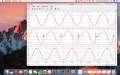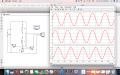# How does a rectifier with a pure inductive load work?

#### crutschow

Joined Mar 14, 2008
25,264
why is the output Voltage still AC? Should the negative half cycle be completely blocked by the diode?
No.
The diode actually blocks the current flow not voltage, so as long as the inductor keeps the current flowing, the diode is forward biased and cannot block the reverse voltage.
It can only do that when the inductor current goes to zero, which is where you see the sudden return to 0V across the inductor.
Why is the current maximum when Input touched zero?
The current keeps increasing as long as there is positive voltage being applied to the inductor (the inductor acts as an integrator).
Thus the current reaches a maximum when the voltage returns to zero and starts to go negative.
This negative voltage will obviously then reduce the current, as observed.

•Devika B S

#### Bordodynov

Joined May 20, 2015
2,644

#### MrAl

Joined Jun 17, 2014
7,757
View attachment 138015
I have simulated the simple inductive load with a diode rectifier in MATLAB. But I am not able to explain the waveforms myself. First, why is the output Voltage still AC? Should the negative half cycle be completely blocked by the diode? Why is the current maximum when Input touched zero?
Hello,

The short answer is that an inductor works like a constant current source for a short time period. The time period depends partly on the value of the inductor and how much current and the value of the energy absorbers in the circuit such as a resistor.

There is another issue that comes up when looking at this using a simulator though,and that is the exponential part of the response. In order to see the steady state response (which is really the more interesting here) there needs to be some resistance in series with the inductor. That helps to ensure that the exponential part of the response dies down quickly so we can see the more typical wave response.

So add a little resistance in series with the inductor, like say 0.1 to 1 ohm.
Also, look at the waveforms both when you have a diode in the circuit and with the diode shorted out and compare. You should see some similarities.

The inductor stores energy unlike a resistor, so it kicks some of that energy back into the circuit at some points in time. During those points in time it keeps some current flowing as it tries to return the energy it stored back into the circuit. You can also make a plot of i^2*L/2 vs time to see this energy level. When the energy level is increasing the inductor is absorbing energy, and when the energy level is decreasing it is putting energy back into the circuit. When the energy level is zero it put all it's stored energy back into the circuit. Since the local monotonicity of the energy is the same as that of the current itself, you can even get a rough idea how the energy is changing just by looking at the current alone.

Last edited:

#### Devika B S

Joined Mar 8, 2017
144
Hello,

So add a little resistance in series with the inductor, like say 0.1 to 1 ohm.Adding 1 ohm resistor isn't making much difference. Maybe the inductance is too high? I have used 100 mH inductance.
Also, look at the waveforms both when you have a diode in the circuit and with the diode shorted out and compare. You should see some similarities.Yes, it is strikingly similar! Almost the same. Just the current waveforms are not rectified in this case. Thats the only major difference.

#### Bordodynov

Joined May 20, 2015
2,644
Look in my example (post # 3). I have an inductance of 10 mH and a resistance of 1 Ohm. The source resistance is also 1 ohm. With an inductive load an additional diode is used, as I have shown.

#### Devika B S

Joined Mar 8, 2017
144
Look in my example (post # 3). I have an inductance of 10 mH and a resistance of 1 Ohm. The source resistance is also 1 ohm. With an inductive load an additional diode is used, as I have shown.
Yes, but where is the exponential part in the steady state. In these type of circuits, there seems to be no steady state. Looks like its going to go on like that.

#### GopherT

Joined Nov 23, 2012
8,012
Yes, but where is the exponential part in the steady state. In these type of circuits, there seems to be no steady state. Looks like its going to go on like that.
Yes, your circuit has no energy storage component so a steady state between AC peaks cannot be achieved. Energy “storage” component would be a magnetic field (a saturated iron core, a capacitor, a battery...). Even the iron core and capacitor would decrease the peaks, not eliminate them. It depends on the load and the frequency.

#### MrAl

Joined Jun 17, 2014
7,757
View attachment 138033

Adding 1 ohm resistor isn't making much difference. Maybe the inductance is too high? I have used 100 mH inductance.
View attachment 138034

Yes, it is strikingly similar! Almost the same. Just the current waveforms are not rectified in this case. Thats the only major difference.
Hi,

The steady state in this kind of circuit is reached when the initial exponential part damps out. This comes from the very first point of turn on only. After that time the circuit will damp out into a more regular behavior.
The resistor helps get to this point faster so if you dont see this behavior with no resistor then that must mean that the simulator is reaching steady state by some other means such as default resistance or purposely solving for the steady state point beforehand. There could be a check box for that somewhere to turn it on or off.
Note that steady state is not necessarily sinusoidal behavior. It is the behavior that occurs after the initial application of energy which occurs at t=0 only has damped out. In other words, it is the time after that extra initial energy has been dissipated. This may or may not turn into a sine wave but will look more regular than at the very start of the simulation. If you dont see that difference then it could be that the simulator is solving for the steady state solution before it actually starts the time solution or that it happens so fast we dont see it until we zoom in to times near t=0.
Also note that with a cosine wave excitation we can reach this point much sooner too.

If you look at the energy you can see it go up when the line puts energy into the inductor, then go down when the inductor puts energy back into the line. When the energy reaches zero the current reaches zero, so when you see the current reach zero that means all the energy has been dumped back into the line. Without the diode the energy still reaches zero, but then the energy starts to increase again as the line goes negative, something we dont see when the diode is present, so the diode limits the time that the inductor can charge to some sub cycle time period. The inductor keeps the diode forward biased until it runs out of energy and then it can no longer keep it biased so the current stays at zero for some time until the line can again reach a point where it can forward bias the diode again.

Last edited:

#### Devika B S

Joined Mar 8, 2017
144
Hi,

The steady state in this kind of circuit is reached when the initial exponential part damps out. This comes from the very first point of turn on only. After that time the circuit will damp out into a more regular behavior.
The resistor helps get to this point faster so if you dont see this behavior with no resistor then that must mean that the simulator is reaching steady state by some other means such as default resistance or purposely solving for the steady state point beforehand. There could be a check box for that somewhere to turn it on or off.
Note that steady state is not necessarily sinusoidal behavior. It is the behavior that occurs after the initial application of energy which occurs at t=0 only has damped out. In other words, it is the time after that extra initial energy has been dissipated. This may or may not turn into a sine wave but will look more regular than at the very start of the simulation. If you dont see that difference then it could be that the simulator is solving for the steady state solution before it actually starts the time solution or that it happens so fast we dont see it until we zoom in to times near t=0.
Also note that with a cosine wave excitation we can reach this point much sooner too.

If you look at the energy you can see it go up when the line puts energy into the inductor, then go down when the inductor puts energy back into the line. When the energy reaches zero the current reaches zero, so when you see the current reach zero that means all the energy has been dumped back into the line. Without the diode the energy still reaches zero, but then the energy starts to increase again as the line goes negative, something we dont see when the diode is present, so the diode limits the time that the inductor can charge to some sub cycle time period. The inductor keeps the diode forward biased until it runs out of energy and then it can no longer keep it biased so the current stays at zero for some time until the line can again reach a point where it can forward bias the diode again.
Even if I zoom it, I still don't get that initial transition current. So I guess by default, its getting ridden off by the Solver. However, I have also managed to get initial transition waveforms earlier (when i was doing a project) by changing some advanced features in Solver parameters.